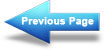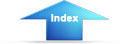Custom SearchSource resistanceMatter, Energy, and ElectricityParallel DC circuitsPOWER TRANSFER AND EFFICIENCY
Maximum power is transferred from the source to the load when the resistance of the load is equal to the internal resistance of the source. This theory is illustrated in the table and the graph of figure 3-36. When the load resistance is 5 ohms, matching the source resistance, the maximum power of 500 watts is developed in the load.

The efficiency of power transfer (ratio of output power to input power) from the source to the load increases as the load resistance is increased. The efficiency approaches 100 percent as the load resistance approaches a relatively large value compared with that of the source, since less power is lost in the source. The efficiency of power transfer is only 50 percent at the maximum power transfer point (when the load resistance equals the internal resistance of the source). The efficiency of power transfer approaches zero efficiency when the load resistance is relatively small compared with the internal resistance of the source. This is also shown on the chart of figure 3-36.

The problem of a desire for both high efficiency and maximum power transfer is resolved by a compromise between maximum power transfer and high efficiency. Where the amounts of power involved are large and the efficiency is important, the load resistance is made large relative to the source resistance so that the losses are kept small. In this case, the efficiency is high. Where the problem of matching a source to a load is important, as in communications circuits, a strong signal may be more important than a high percentage of efficiency. In such cases, the efficiency of power transfer should be only about 50 percent; however, the power transfer would be the maximum which the source is capable of supplying.

You should now understand the basic concepts of series circuits. The principles which have been presented are of lasting importance. Once equipped with a firm understanding of series circuits, you hold the key to an understanding of the parallel circuits to be presented next.

A circuit has a source voltage of 100 volts and two 50-ohm resistors connected in series. If the reference point for this circuit is placed between the two resistors, what would be the voltage at the reference point?

If the reference point in question 25 were connected to ground, what would be the voltage level of the reference point?

What is an open circuit?

What is a short circuit?

Why will a meter indicate more voltage at the battery terminal when the battery is out of a circuit than when the battery is in a circuit?

What condition gives maximum power transfer from the source to the load?

What is the efficiency of power transfer in question 30?

A circuit has a source voltage of 25 volts. The source resistance is 1 ohm and the load resistance is 49 ohms. What is the efficiency of power transfer?Integrated Publishing, Inc. - A (SDVOSB) Service Disabled Veteran Owned Small Business